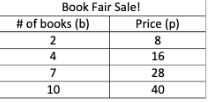There Is No Such Thing As A Second Impression.
WELCOME
CALL (917) 748-9089
• No products in the cart.
Top

### TACHS Math Quiz #9

October 1, 2023

Math Test: TACHS Math Quiz #9

What class are you in?*

MULTIPLE CHOICE. Choose the one alternative that answers the question.

1) 6b = 30

2) 12 + q = 56

3)  Simplify by combining like terms: 7v + 2 + 12 + 2v

4 ) 9x —  7 = -7

5) 8n + 7 = 31

6) 2(-x — 4) = 4x + 16

7) What is the multiplicative inverse of ⅔?

8) The temperature was 37 degrees on January 3rd.  On January 4th, it dropped 45 degrees.  What was the temperature on January 4th?

9) Simplify the expression

-4(2 - 3t)

10) The local elementary school is having a special sale at the book fair.  The table shows the price breakdown.  Which equation could be used to determine the price per book?11) Subtract (-14) —  (-18)

12)  Choose the true statement.

13)  Bryan gets on an elevator at the 35th floor. The elevator goes down 17 floors then up 9 floors. At what floor does it finally stop?